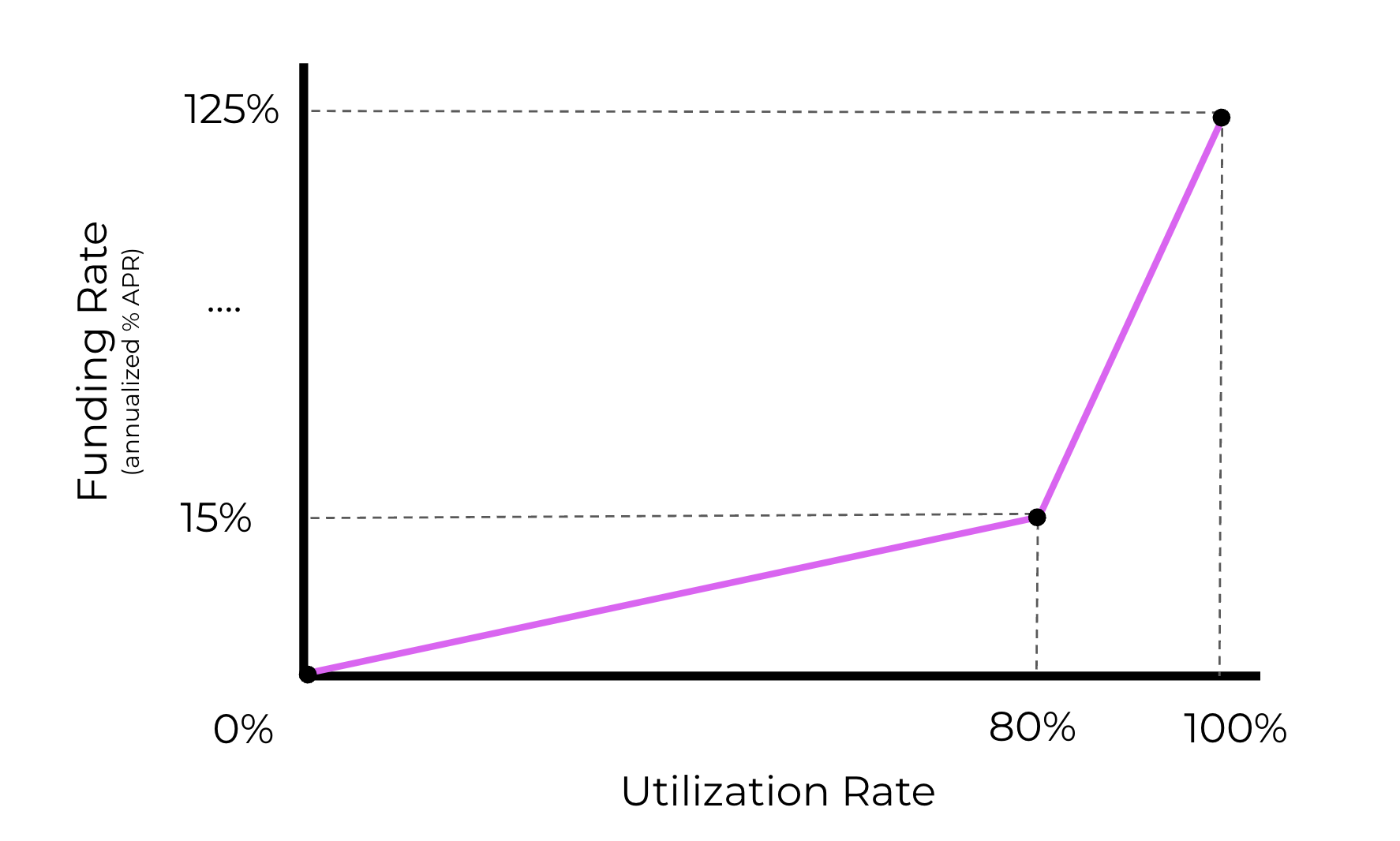Comment on page

# Interest Rate

In order to keep the maker side of the market incentivized even when we have relatively balanced long and shorts, markets can charge an interest rate. This is meant to measure the delta-neutral capital costs of the makers, whereas funding covers their net exposure.

## Utilization

The interest rate is determined by a utilization curve and is given to all maker positions. In order to cap the spread, if there is a greater amount of maker position than the sum of the long and short positions, the interest rate is pro-rata discounted. Utilization is determined by measuring the greater side against the sum of the maker and lesser side. Intuitively, the interest rate is a funding spread used to incentivize idle liquidity.In this example, the utilization is 10 / (5 + 6) or 91%. Both longs and shorts pay their pro-rata portion of the overall market interest to the maker side of the market.

## Utilization Curve

On market creation, the market operator selects a utilization function which defines the interest rate paid to makers at every level of utilization, allowing each market to have have a different delta-neutral cost of capital. Perennial uses Compound-style utilization curves that are algorithmic & continuous.
Jump-rate Curves
The search for a perfect curve is an ongoing battle, and these curves will need to be actively managed.
In JumpRate curves, the curve is parameterized to slowly increase in a linear fashion as utilization climbs to the target utilization at which it reaches its target rate. Then, beyond this target utilization, the rate increases rapidly to incentivize the market to rebalance back to the target utilization/rate.
To construct a jump rate curve, 4 parameters are needed:
Min Rate: Lowest acceptable rate
Max Rate: highest rate traders would be. willing to pay (sufficiently high to balance liquidity during times of high utilization)
Target Rate: The rate when the market is at equilibrium
Target Utilization: the equilibrium utilization level
$\begin{cases} minRate + Utilization * (targetRate - minRate) & Utilization \leq targetUtilization \\ targetRate+ (Utilization - targetUtilization) *(maxRate-targetRate) & Utilization > targetUtilization \end{cases}$
Example of a JumpRate curve with the following parameters:
MinRate
MaxRate
TargetUtilization
TargetRate
0%
125%
80%
15%